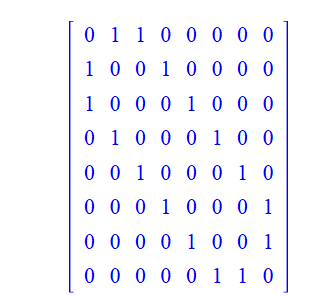# Question:what is the advantage of option " bits " of NonIsomorphicGraphs?

## Question:what is the advantage of option " bits " of NonIsomorphicGraphs?

Maple 2021

Recently I saw a heated discussion on this post：https://www.mapleprimes.com/questions/233026-Write-Output-Of-For-Loop-To-txt-File

In Carl Love  's answer, I felt the power of adjacency matrix of graph. I carefully read maple's function to generate non IsomorphicGraphs:  NonIsomorphicGraphs. There was one passage that I thought was really interesting.

selectform = graph, adjacency, or bits
This specifies the form used for a graph when it is being passed to the select procedure. The forms are identical to those for outputform, but note that adjacency and bits are the most efficient forms, while graph is only reasonable for a relatively small number of comparison operations (1000 or less), as it needs to form a Graph for every comparison. Note also that when selectform=adjacency the same read-only Matrix is internally used to pass the data for every call to reduce overhead. This is not possible with a Graph structure.

I never quite understood what the advantage of this bits was. At least according to the help documentation, we have  to do many works to do to convert this into a adjacency matrix of graph.

```[NonIsomorphicGraphs(8, output = graphs, restrictto = regular)]

bit := Vector[row](Bits:-Split(210256131, bits = (8*(8 - 1))/2));
M := Matrix(8, 8, shape = symmetric, datatype = integer);
M[1, 2 .. 8] := bit[1 .. 7];

M[2, 3 .. 8] := bit[8 .. 13];

M[3, 4 .. 8] := bit[14 .. 18];
M[4, 5 .. 8] := bit[19 .. 22];

M[5, 6 .. 8] := bit[23 .. 25];

M[6, 7 .. 8] := bit[26 .. 27];
M[7, 8 .. 8] := bit[28 .. 28];
M;```From adjacency matrix of graph,  we can diractly get a lot of information about the graph, like edges, and the number of closed walks of a particular length, but from bits , what imformation of graph we can get?

If we have to convert it to an adjacency matrix fist, maybe it's a little bit more complicated from above long codes. At least there isn't a more efficient way to convert?﻿